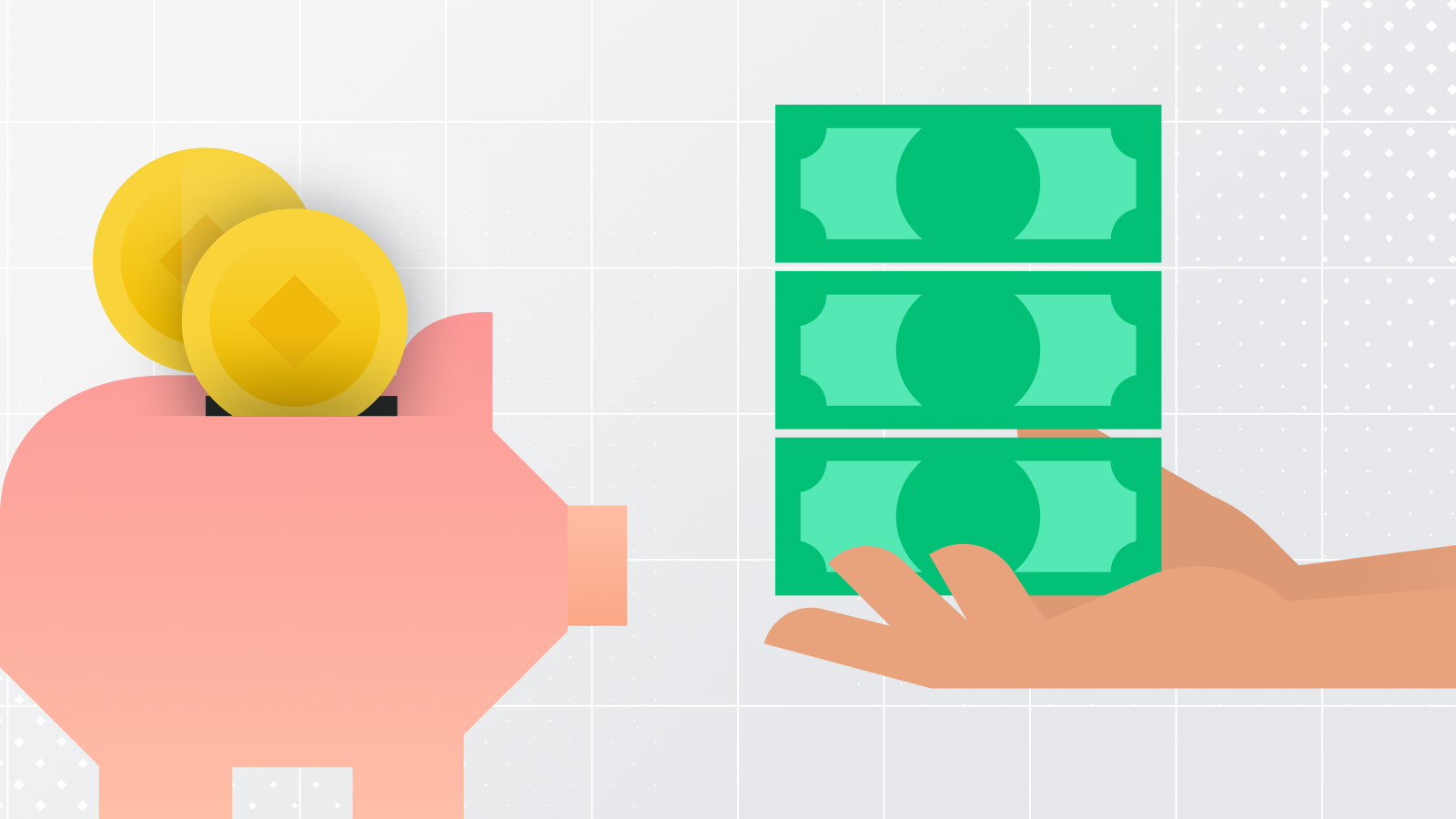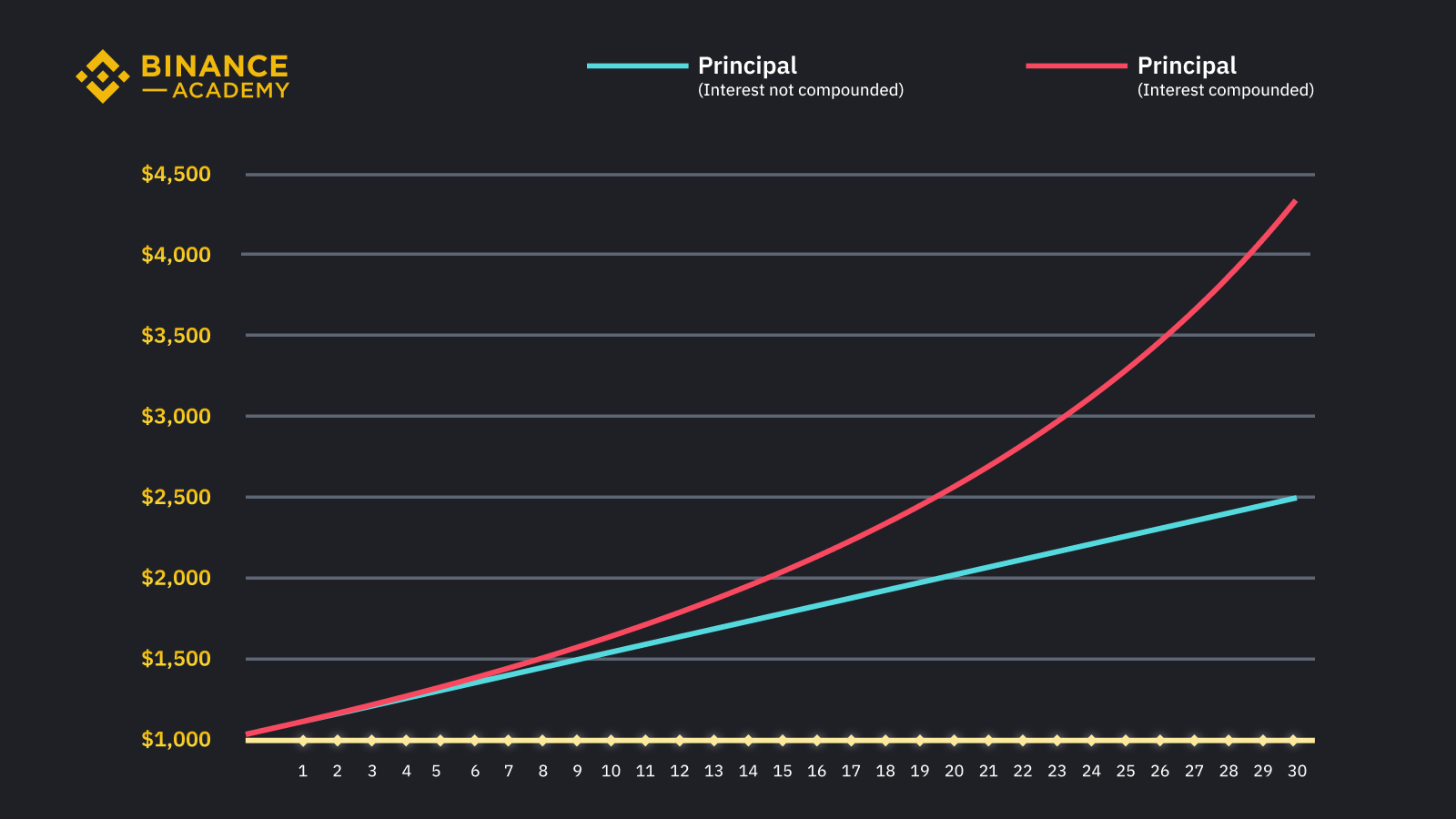Startseite
Artikel
APY vs APR: What’s the Difference?

# APY vs APR: What’s the Difference?

Beginner
Veröffentlicht Jul 27, 2022Aktualisiert Mar 21, 2023
5m

TL;DR

You have likely seen these two similar-sounding terms, APY and APR, when looking into decentralized finance (DeFi) products.

APY, or annual percentage yield, incorporates interest compounded quarterly, monthly, weekly, or daily, while APR, or annual percentage rate, doesn’t. This simple distinction can make a significant difference to the calculations for returns over a period of time. It is therefore important to understand how these two metrics are calculated and what it means for the returns that you can earn on your digital funds.## APR vs. APY

APR and APY are both fundamental for the purposes of personal finance. Let’s start with the simpler term, annual percentage rate (APR). It is the interest rate a lender earns on their money — and that a borrower pays for using it — over one year’s time.

For example, if you put \$10,000 into a bank savings account with a 20% APR, you will get \$2,000 in interest after one year. Your interest is calculated by multiplying the principal amount (\$10,000) and the APR (20%). So, after one year, you will have a total of \$12,000. After two years, your capital will amount to \$14,000. After three years, you will have \$16,000, and so on.

Before getting into annual percentage yield (APY), let’s first understand what compound interest is. Put simply, it means earning interest on the previous interest. In the example above, if the financial institution pays interest to your account monthly, your balance will look different during each of the twelve months of the year.

Instead of getting \$12,000 at the end of the 12th month, you will receive some interest each month. That interest is added to the principal sum of your deposit, and the sum on which you earn interest goes up as the months go by. Each month, you will have more money earning interest. This effect is called compounding.

Let’s say that you put \$10,000 into a bank account with a 20% APR, with the interest compounding monthly. Without getting into the complicated math, you will get \$12,194 at the end of one year. That is \$194 more in interest earned simply by adding the effect of compound interest. How much interest you’d earn with the exact 20% APR but with interest compounded daily? That would give you \$12,213.

The power of compounding is more impressive over more extended periods. After three years, you would end up with \$19,309 with the same 20% APR product with daily compounding. That’s \$3,309 more interest earned than that same 20% APR product without compounding.By simply incorporating compound interest, you’d earn a lot more on your money. Notice also that the interest differs according to the compounding frequency. You earn more when the compounding is more frequent. Daily compounding will give you more interest than monthly compounding.

How do you calculate how much you can earn when a financial product offers compound interest? That’s where annual percentage yield (APY) comes in. You can use a formula to convert an APR to APY depending on the frequency of compounding. A 20% APR with monthly compounding equals 21.94% in APY. With daily compounding, it would equal 22.13% APY. These APY numbers represent the annualized interest returns you earn after incorporating compound interest.

In sum, APR (annual percentage rate) is a simpler and more static metric: It’s always quoted as a fixed yearly rate. But APY (annual percentage yield) incorporates interest earned on interest, or compound interest. It changes according to the compounding frequency. One way to memorize the difference is to remember that “yield” has five letters (one more letter than “rate”) and also represents the more complex concept (and greater earnings).

## How to compare different interest rates?

From the example above, you can see that more interest can be earned when interest is compounded. Different products may present their rates as either APR or APY. Because of this disparity, it’s essential to use the same term for comparison. Be mindful when you compare products,  as you may be comparing apples to oranges.

Products with a higher APY will not necessarily yield more interest than those with a lower APR. You can easily convert APR and APY using online tools if you know the frequency of compounding.

The same goes for DeFi and other kinds of crypto products. When looking at products that may advertise using crypto APY and APR, such as crypto savings and staking, make sure to convert them so that you can compare apples to apples.

Further, when comparing two DeFi products with APY, make sure that they have the same compounding periods. If they have the same APR, but one compounds monthly and the other daily, then the one that compounds daily may earn you more crypto interest.

Another important point to note is what APY means in relation to the specific crypto product you are reviewing. Some product collaterals use the term “APY” to refer to the rewards that one can earn in cryptocurrency over the selected timeframe, and not the actual or predicted returns/yield in any fiat currency. This is an important distinction to appreciate because crypto asset prices can be volatile, and the value of your investment (in fiat terms) may go down or up. If the crypto asset prices fall drastically, the value of your investment (in fiat terms) may still be lower than the original fiat amount you had invested, even if you continue to earn an APY in crypto assets. It is therefore important that you review the relevant product terms and conditions carefully, and do your own research, to fully understand the investment risks involved and what APY means in that specific context.## Closing thoughts

APR and APY may seem confusing initially, but it’s easy to tell one from another by remembering that annual percentage yield (APY) is the more complex metric incorporating compound interest. Because of the effect of earning interest on interest, APY is always a higher number when interest is compounded more frequently than once a year. The bottom line is always to check which rate you are looking at when calculating the interest you’d earn.